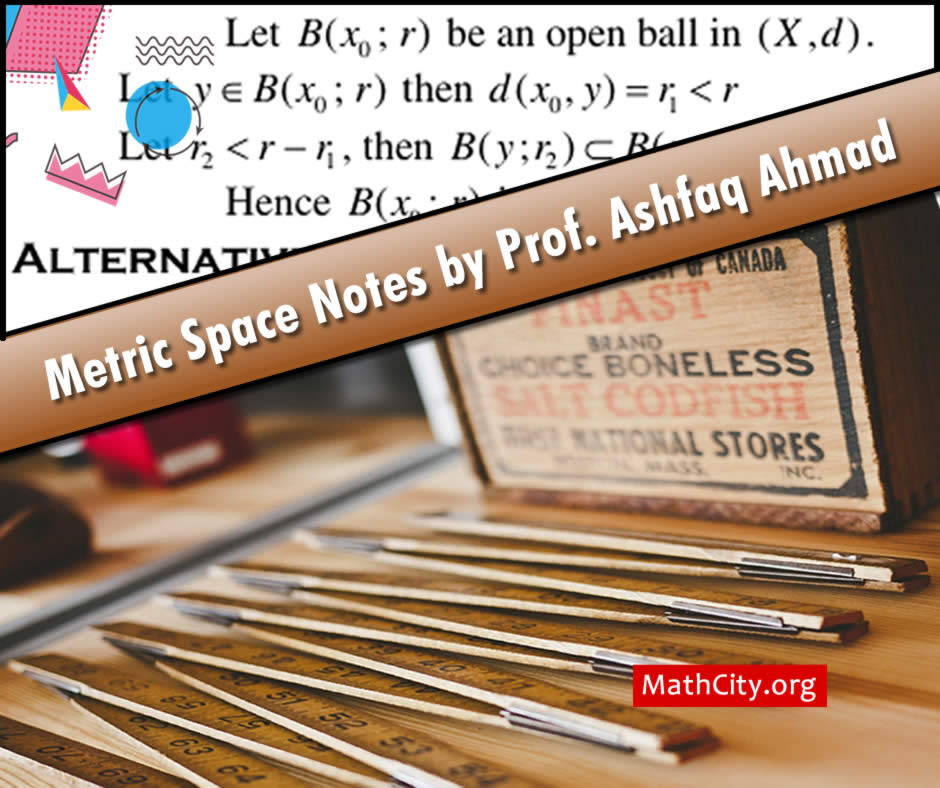# Metric Spaces (Notes)These are updated version of previous notes. Many mistakes and errors have been removed. These notes are collected, composed and corrected by Atiq ur Rehman, PhD. These are actually based on the lectures delivered by Prof. Muhammad Ashfaq (Ex HoD, Department of Mathematics, Government College Sargodha).

These notes are very helpful to prepare a section of paper mostly called Topology in MSc for University of the Punjab and University of Sargodha. These are also helpful in BSc.

 Name Metric Spaces (Notes) - Version 2 Atiq ur Rehman, PhD Prof. Muhammad Ashfaq 24 pages PDF 275KB
• Metric Spaces and examples
• Pseudometric and example
• Distance between sets
• Theorem: Let $(X,d)$ be a metric space. Then for any $x,y\in X$, $$\left| {\,d(x,\,A)\, - \,d(y,\,A)\,} \right|\,\, \le \,\,d(x,\,y).$$
• Diameter of a set
• Bounded Set
• Theorem: The union of two bounded set is bounded.
• Open Ball, closed ball, sphere and examples
• Open Set
• Theorem: An open ball in metric space X is open.
• Limit point of a set
• Closed Set
• Theorem: A subset A of a metric space is closed if and only if its complement $A^c$ is open.
• Theorem: A closed ball is a closed set.
• Theorem: Let (X,d) be a metric space and $A\subset X$. If $x \in X$ is a limit point of A. Then every open ball $B(x;r)$ with centre x contain an infinite numbers of point of A.
• Closure of a Set
• Dense Set
• Countable Set
• Separable Space
• Theorem: Let (X,d) be a metric space, $A \subset X$ is dense if and only if A has non-empty intersection with any open subset of X.
• Neighbourhood of a Point
• Interior Point
• Continuity
• Theorem: $f:(X,d)\to (Y,d')$ is continuous at $x_0\in X$ if and only if $f^{-1}(G)$ is open is X. wherever G is open in Y.
• Convergence of Sequence
• Theorem: If $(x_n)$ is converges then limit of $(x_n)$ is unique.
• Theorem: (i) A convergent sequence is bounded. (ii) ii) If ${x_n}\to x$ and ${y_n}\to y$ then $d(x_n,y_n)\to d(x,y)$.
• Cauchy Sequence
• Theorem: A convergent sequence in a metric space (X,d) is Cauchy.
• Subsequence
• Theorem: (i) Let $(x_n)$ be a Cauchy sequence in (X,d), then $(x_n)$ converges to a point $x\in X$ if and only if $(x_n)$ has a convergent subsequence $\left(x_{n_k}\right)$ which converges to $x\in X$.
• (ii) If $(x_n)$ converges to $x\in X$, then every subsequence $\left(x_{n_k}\right)$ also converges to $x\in X$.
• Theorem: Let (X,d) be a metric space and $M \subseteq X$. (i) Then $x\in\overline{M}$ if and only if there is a sequence $(x_n)$ in M such that $x_n\to x$. (ii) If for any sequence $(x_n)$ in M, ${x_n}\to x\quad\Rightarrow\quad x\in M$, then M is closed.
• Complete Space
• Subspace
• Theorem: A subspace of a complete metric space (X,d) is complete if and only if Y is closed in X.
• Nested Sequence
• Theorem (Cantor’s Intersection Theorem): A metric space (X,d) is complete if and only if every nested sequence of non-empty closed subset of X, whose diameter tends to zero, has a non-empty intersection.
• Complete Space (Examples)
• Theorem: The real line is complete.
• Theorem: The Euclidean space $\mathbb{R}^n$ is complete.
• Theorem: The space $l^{\infty}$ is complete.
• Theorem: The space C of all convergent sequence of complex number is complete.
• Theorem: The space $l^p,p\ge1$ is a real number, is complete.
• Theorem: The space C[a, b] is complete.
• Theorem: If $(X,d_1)$ and $\left(Y,d_2\right)$ are complete then $X\times Y$ is complete.
• Theorem: $f:\left(X,d\right)\to\left(Y,d'\right)$ is continuous at $x_0\in X$ if and only if $x_n\to x$ implies $f(x_n)\to f(x_0)$.
• Rare (or nowhere dense in X)
• Meager (or of the first category)
• Non-meager (or of the second category)
• Bair’s Category Theorem: If $X\ne\phi$ is complete then it is non-meager in itself “OR” A complete metric space is of second category.

• notes/metric-spaces-notes